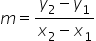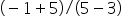Mathematics
Easy

Question

# Write the equation in point-slope form for the line that contains the points(3, -5) and (5, - 1)

## y - 5 = 2(x + 3)y + 5 = 2(x - 3)y - 3 = 2(x + 5)y + 3 = 2(x - 5)Hint:

## The correct answer is: y + 5 = 2(x - 3)

### Given The points (3, -5) and (5, - 1).Step1 : calculating slopeSlope of a line that passes through (x1,y1), (x2, y2)==2Step 2 : making point slope formPoint-slope form of equation of a line is y - y1 = m (x - x1)Substitute point (3, -5) and m value.y + 5 = 2 (x -3)y + 5 = 2 (x -3) , is desired equation .

First calculate the slope making use of two points
and then using slope and point construct the equation of line.

### Related Questions to study#### With Turito Foundation.#### Get an Expert Advice From Turito.